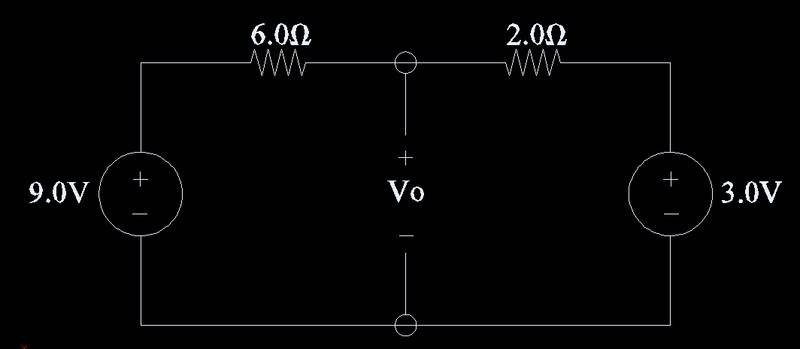# Kirchoffs Voltage Law

## Homework Statement

Determine Vo in the circuit below:## Homework Equations

V1 +V2+V3+...Vn=0

## The Attempt at a Solution

I think this should be solved using KVL, but I have no idea on where to exactly start.

By looking at the problem, im thinking it will be 6.0V because 9.0V-3.0V=6.0V,
but dont think thats right.

Any help would be greatly appreciated

Last edited:

## Answers and Replies

Realise that you've got a potential divider across the two resistors, with an overall p.d. of 6V. 6V split in 6:2... so what's the voltage in the middle?

Realise that you've got a potential divider across the two resistors, with an overall p.d. of 6V. 6V split in 6:2... so what's the voltage in the middle?

Im kind of lost on what you just said, could you elaborate a little?

Andrew

Calculate the current through the two resistors and then the voltage drop across the 6 ohm resistor.

kuba
Potential divider...

Well, what you have is two resistors in series, which is a potential divider.
In this case one end of the divider is not attached to the "ground", but to some other voltage. You can apply usual potential divider equations, just remember to add your reference voltage (3.0V) to it.

The difference between voltages at ends of the divider is 6V.

Voltage in the middle, referenced to 3V (right end of the divider) is

$$V_{m(3V)}=6V\frac{2.0\Omega}{6.0\Omega+2.0\Omega}=1.5V$$

This is referenced to the right end of the divider. If you want it referenced to "ground", i.e. the $$V_o$$ voltage, just add 3V to it.

You can also reference everything to 9V. Then the divider is powered from -6V, and

$$V_{m(9V)}=-6V\frac{6.0\Omega}{6.0\Omega+2.0\Omega}=-4.5V$$

This is referenced to the left end of the divider. To reference it to "ground", add 9V to it.

You will obtain the same answer either way.

The resistive divider "equation" I've used above can be derived from Ohm's and Kirchhof's laws.

Well, what you have is two resistors in series, which is a potential divider.
In this case one end of the divider is not attached to the "ground", but to some other voltage. You can apply usual potential divider equations, just remember to add your reference voltage (3.0V) to it.

The difference between voltages at ends of the divider is 6V.

Voltage in the middle, referenced to 3V (right end of the divider) is

$$V_{m(3V)}=6V\frac{2.0\Omega}{6.0\Omega+2.0\Omega}=1.5V$$

This is referenced to the right end of the divider. If you want it referenced to "ground", i.e. the $$V_o$$ voltage, just add 3V to it.

You can also reference everything to 9V. Then the divider is powered from -6V, and

$$V_{m(9V)}=-6V\frac{6.0\Omega}{6.0\Omega+2.0\Omega}=-4.5V$$

This is referenced to the left end of the divider. To reference it to "ground", add 9V to it.

You will obtain the same answer either way.

The resistive divider "equation" I've used above can be derived from Ohm's and Kirchhof's laws.

Thanks for the replies! My only question is that we havnet discussed potential dividers yet. Is this the only way of doing this or is it possible in some other way?

Thanks for the replies! My only question is that we havnet discussed potential dividers yet. Is this the only way of doing this or is it possible in some other way?

As I said in my previous post, calculate the current through the 2 resistors:
$$i = \frac {9-3}{6 + 2}$$
The voltage is:
$$V_0 = 9 - 6 i$$

So using the potential divider formula, this is what I came to:

Vo=(V1(R2/(R1+R2))+V2

Plugging that in it gives me 5.25V.

As I said in my previous post, calculate the current through the 2 resistors:
$$i = \frac {9-3}{6 + 2}$$
The voltage is:
$$V_0 = 9 - 6 i$$

Doing it this way I get i=6/8=.75A
Vo=9-6(.75)=4.5V

Im thinking that this way is correct.

Thanks! Sorry I didnt even notice your post.